# OpenLearn Geometry¶

This notebook recreates some of the content featured in the OpenLearn course Geometry.

The notebook includes several hidden code cells that generate the a range of geometric figures.

To render the images, go to the Cell menu and select Run All.

To view/hide the code used to generate the figures, click on the Hide/Reveal Code Cell Inputs button in the notebook toolbar.

To make changes to the diagrams, click in the appropriate code input cell, make your change, and then run the cell using the Run Cell ("Play") button in the toolbar or via the keyboard shortcut SHIFT-ENTER.

Entering Ctrl-Z (or CMD-Z) in the code cell will undo your edits...

## Angles¶

The Try some yourself activity includes the following shape.

In :
%load_ext tikz_magic

In :
%%tikz
\usetikzlibrary{positioning}

\coordinate (A) at (0,0) ;
\coordinate (B) at (5,0.3) ;
\coordinate (C) at (4.5,-2) ;
\coordinate (D) at (2,-3) ;

\draw (A) node[left]{A}  -- (B)node[right]{B};
\draw  (B) -- (C)node[right]{C};
\draw  (C)  -- (A) ;
\draw  (A)  -- (D) node[below]{D};
\draw  (D) -- (C);

\begin{scope}
\clip (B) -- (A) -- (C);
\draw (A) circle[radius=1.1];
\end{scope}
\node at (A)[below right=-0.05 and 0.5 ] {$\alpha$};

\begin{scope}
\clip (C) -- (A) -- (D);
\draw (A) circle[radius=1.1];
\end{scope}
\node at (A)[below right=0.3 and 0.3 ] {$\gamma$};

\begin{scope}
\clip (B) -- (C) -- (A);
\draw (C) circle[radius=1];
\end{scope}
\node at (C)[above left=0.25 and 0.2 ] {$\beta$};

\begin{scope}
\clip (A) -- (C) -- (D);
\draw (C) circle[radius=1];
\end{scope}
\node at (C)[left=0.4 ] {$\delta$};

Out: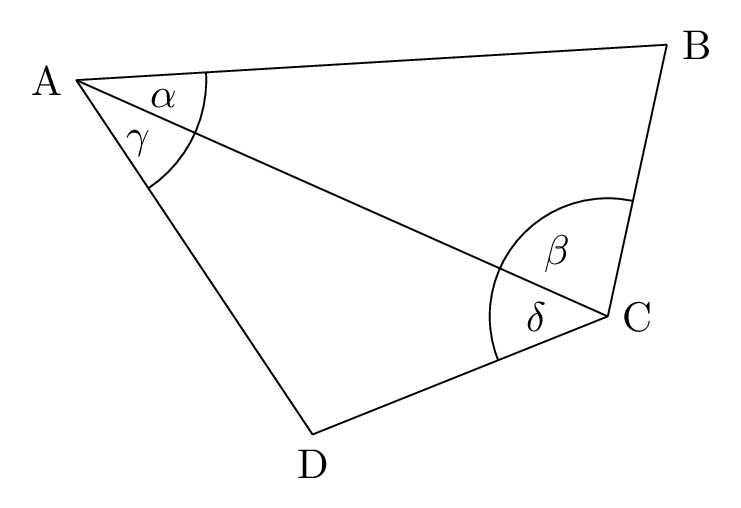## Geometric shapes – circles¶

Original link

All circles are the same shape – they can only have different sizes.

In a circle, all the points are the same distance from a point called the centre. The centre is often labelled with the letter O.

In :
%%tikz
%https://tex.stackexchange.com/a/223219

\def\radius{2}% radius of the circle
\def\tilt{30}% angle for the arc

%origin
\coordinate (O) at (0,0) ;

% Circle
\draw (O) circle[radius=\radius] node[font=\tiny, right]{{\em{O}} (Centre)};

%Centre point
\draw[black,fill=black] (0,0) circle [radius=0.03];

%Diameter
%latex-latex defines the 'latex' arrow head at each end of the line
\draw[latex-latex ] (\tilt:\radius) -- (180+\tilt:\radius)
node[font=\tiny,above, midway,rotate=\tilt]{Diameter};

%Radius
\draw[latex-latex ] (O) -- (\tilt+270:\radius)
node[font=\tiny,above, midway, above right]{Radius};

%Circumference
\draw (\tilt+30:\radius) -- +(0.5,1) -- +(0.75,1) node [right]{\tiny{Circumference}} ;

Out: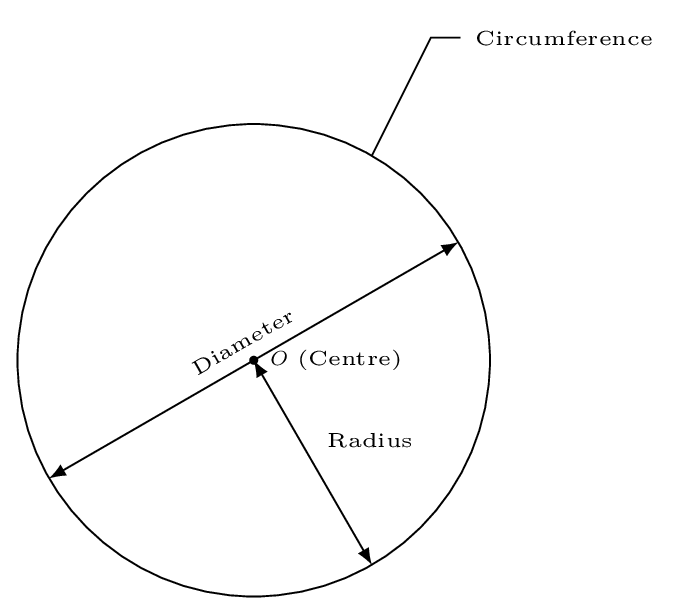The outside edge of a circle is called the circumference. A straight line from the centre to a point on the circumference is called a radius of the circle (the plural of radius is radii).

A line with both ends on the circumference and passing through the centre is called a diameter. Any diameter cuts the circle into two halves called semicircles.

In :
%%tikz
% Define radius
\def\radius{1.5}

%origin
\coordinate (O) at (0,0) ;
\path (O) +(\radius,0) coordinate (arcOrigin);

%Draw dashed semi-circle
\draw [dashed] (arcOrigin) arc(0:-180:\radius);

%Draw grey semicircle -  the -- cycle component closes the shape
\draw [fill={black!30}] (arcOrigin) arc(0:180:\radius)
%Close the figure and add a diameter label
-- cycle node [font=\tiny,below,midway]{Diameter};

%Centre point
\draw[black,fill=black] (O) circle [radius=0.03];

%Circumference label - build a relative line to connent circumference and label
\draw (30:\radius) -- ++(0.5,1) -- +(0.25,0) node [font=\tiny,right,
align=left]{Half the \\ circumference} ;

Out: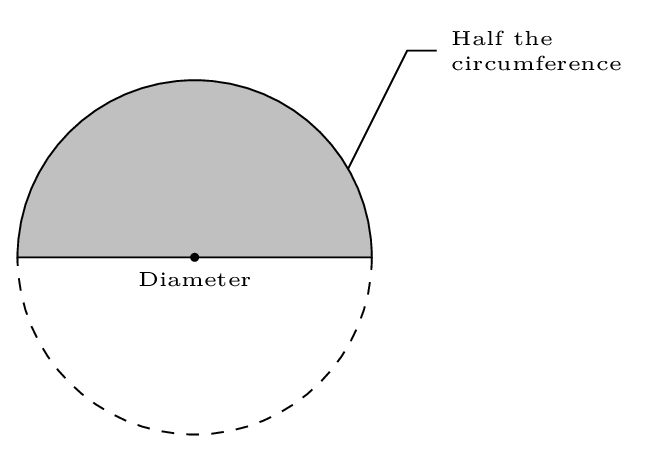In the circle below, the lines labelled OA, OB, OC, OD and OE are all radii, and AD and BE are diameters. The points A, B, C, D and E all lie on the circumference.

In :
%%tikz

\def\radius{2}% radius of the circle

%origin
\coordinate (O) at (0,0) ;

% Circle
\draw (O) circle[radius=\radius] node[font=\tiny, left]{\em{O}};

%Centre point
\draw[black,fill=black] (0,0) circle [radius=0.03];

\draw (O) -- (125:\radius) node[font=\tiny,above]{A};
\draw (O) -- (60:\radius) node[font=\tiny,above right]{B};
\draw (O) -- (0:\radius) node[font=\tiny,right]{C};
\draw (O) -- (-50:\radius) node[font=\tiny,right]{D};
\draw (O) -- (-140:\radius) node[font=\tiny,left]{E};

Out: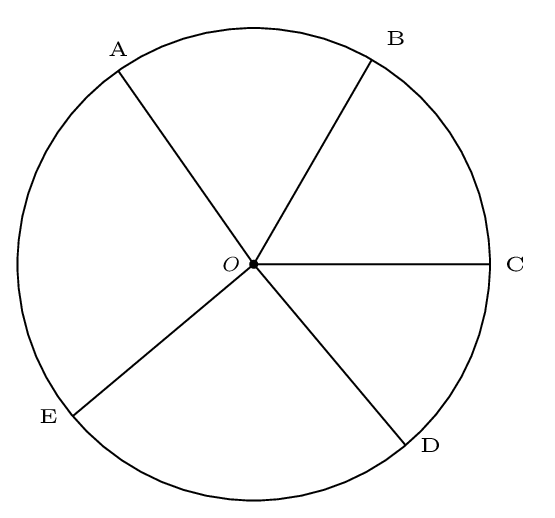Although the terms ‘radius’, ‘diameter’ and ‘circumference’ each denote a certain line, these words are also employed to mean the lengths of those lines. So it is common to say, for example, ‘Mark a point on the circumference’ and ‘The circumference of this circle is 7.3 cm’. It is obvious from the context whether the line itself or the length is being referred to.

### Extra Examples¶

Handy fragments from Stack Overflow..

In :
%%tikz
%https://tex.stackexchange.com/a/197711
\path (120:3) coordinate (A) (0:3) coordinate (B) (0:0) coordinate (C);
\draw (A)
-- (B) node [at start, above left] {$A$} node [midway, above] {$c$}
-- (C) node [at start, right]      {$B$} node [midway, below] {$a$}
-- (A) node [at start, below]      {$C$} node [midway, below] {$b$}
-- cycle;
\draw [dashed] (A) |- (C) node [midway, below left] {$P$};

%The -- (60:.6) element adds the tick to the angle
\draw (0:.5) arc (0:120:.5) (60:.4) -- (60:.6);

Out: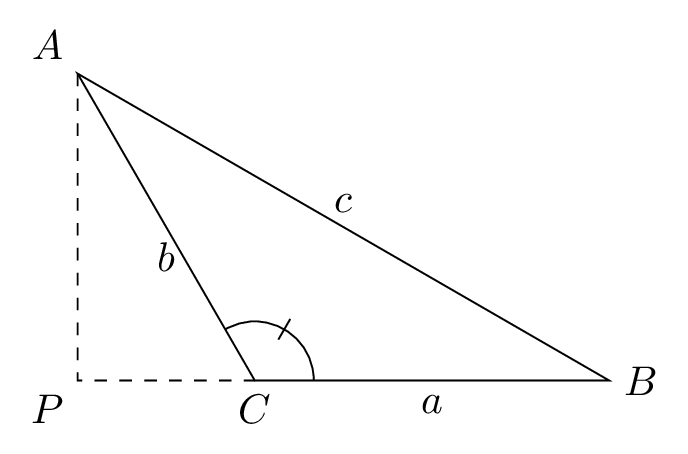In [ ]:


In :
%%tikz --no-wrap
%via https://tex.stackexchange.com/a/397793/151162
\usetikzlibrary{angles,quotes}

\begin{tikzpicture}[x=4cm, y=4cm, axes/.style={thin, gray, ->},
dot/.style={.. dot={#1:0:;}},
.. dot/.style args={#1:#2:#3;}{insert path={
coordinate (#1)
node [circle, fill, inner sep=0, minimum size=2pt,label=#2:#1]{}
}}]
\clip (-0.25, -0.25) rectangle (1.5,1.5);
\draw[axes] (-1.2,0) -- (1.2,0) node[right] {$x$};
\draw[axes] (0,-1.2) -- (0,1.2) node[above] {$y$};
\def\a{40}
\path
(0,0)  [dot=O:225]
(0:1)  [dot=A:315]
(\a:1) [dot=B:90]
(0:cos \a) [dot=C:270]
(\a:sec \a) [dot=D]
(1, cosec \a-cot \a) [dot=E];
\draw (O) circle[radius=1];
\draw (O) -- (B) -- (C);
\draw (B) -- (D) -- (A);
\draw [dashed] (B) -- (A);
\draw [dashed] (B) -- (E);
\pic ["$\theta$", draw, ->, angle radius=1cm] {angle=C--O--B};
\path (O) -- (B) node [midway, above] {$1$};
\path (O) -- (C) node [midway, below] {$\cos\theta$};
\end{tikzpicture}

Out: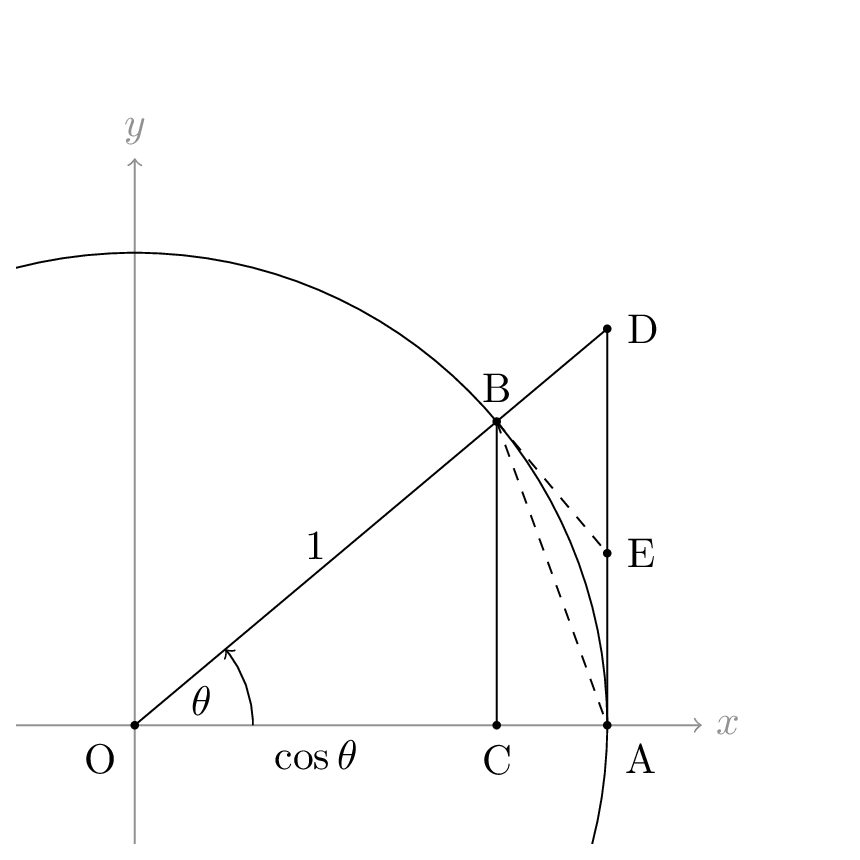In [ ]: